## Saturday, December 29, 2012

### More Equations Involving Natural Numbers - Part 2

To my readers, I get such pleasure from writing on this blog, and fully appreciate each and every one of you. I appreciate the comments. Thank you everyone.

Here's to a Happy and Safe New Years Celebration and to a kick ass 2013!

Onto today's post...

Let A, B, X ∈ N. (Where N is the set of natural numbers (counting numbers). The set can include or not include zero, depending in the definition used. For this blog entry, zero is included.)

One great thing about Casio graphing calculators is that you can make mini-programs. On the current generation (fx-9860g II, Prizm, this includes the older models), within the RUN/MAT mode set the calculator in Linear Input mode. Type each command as you would entering commands in Program mode. Separate each command with a colon (:). I will post screen shots from a Casio Prizm to demonstrate the search for solutions.

For each case, I will present base cases (A = 0, B = 0, and A = B = 0).

(I) A² + B² = X³

Let A = 0, then B² = X³. Likewise, if B = 0, then A² = X³. Without loss of generality, let A = 0.

Then B² = X³. Note that
64 = 4³ = 8² ⇒ B=8, X=4
216 = 6³ = 16² ⇒ B=16, X=6
4096 = 16³ = 64² ⇒ B=64, X=16

More solutions are possible.

Now let A ≠ 0 and B ≠ 0. Then solving for A would yield A = √(X³ - B²). Let B range from 1 to int(√X). Note that int is the integer function. The integer function returns the integer part of a number. Example: int(π) = 3.

Below is the instructions on how I found possible solutions on the Casio Prizm. Note I am looking for integer solutions, ignoring all solutions that were not natural numbers.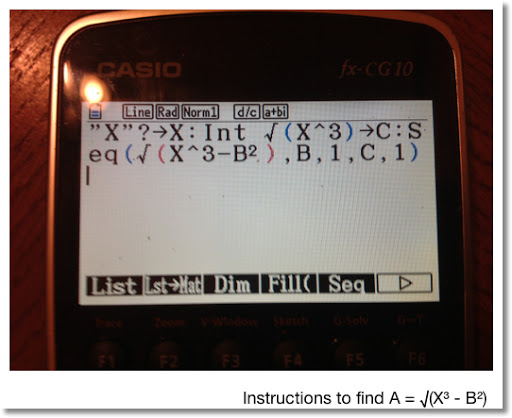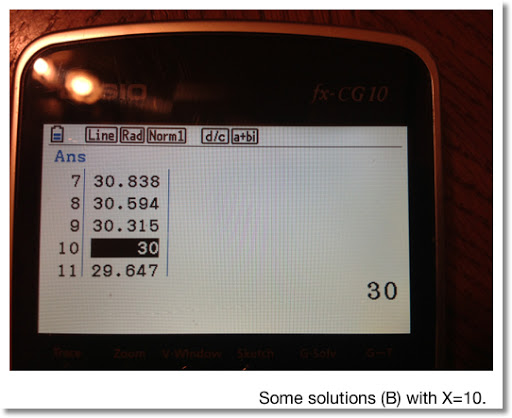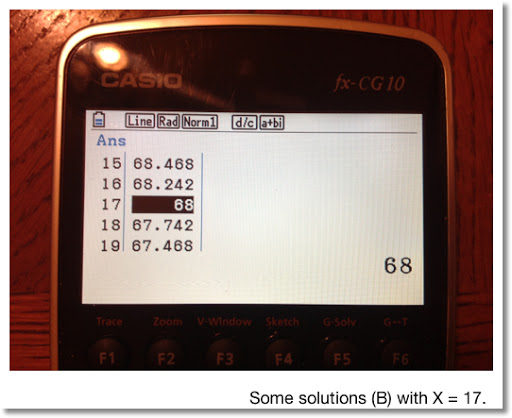Some solutions with X = 1 to 20... (A and B can be interchanged for (I))

(X=2, A=2, B=2)
8 = 2³ = 2² + 2² = 4 + 4

(X=5, A=2, B=11)
125 = 5³ = 2² + 11² = 4 + 121

(X=5, A=5, B=10)
125 = 5³ = 5² + 10² = 25 + 100

(X=8, A=16, B=16)
216 = 8³ = 16² + 16² = 256 + 256

(X=10, A=10, B=30)
1000 = 10³ = 10² + 30² = 100 + 300

(X=10, A=18, B=26)
1000 = 10³ = 18² + 36² = 324 + 676

(X=13, A=9, B=46)
2197 = 13³ = 9² + 46² = 81 + 2116

(X=13, A=26, B=39)
2197 = 13³ = 26² + 39² = 676 + 1521

(X=17, A=17, B=68)
4913 = 17³ = 17² + 68² = 289 + 4624

(X=17, A=47, B=52)
4913 = 17³ = 47² + 52² = 2209 + 2704

(X=18, A=54, B=54)
5832 = 18³ = 54² + 54² = 2916 + 2916

(X=20, A=16, B=88)
8000 = 20³ = 16² + 88² = 256 + 7744

(X=20, A=40, B=80)
8000 = 20³ = 40² + 80² = 1600 + 6400

(II) A² + B³ = X³

If A=0, then B=X, because B³ = X³. For B, X ∈ N.

If B=0, then A² = X³. Some solutions are:
A=1, X=1
A=8, X=4

Let A ≠ 0 and B ≠ 0. Solving for A yields A = √(X³ - B³). Here is the algorithm I used with the my Casio Prizm: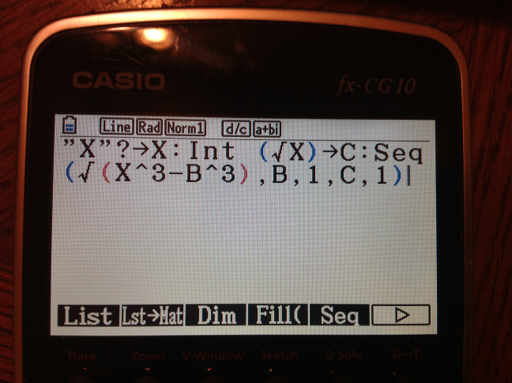Looking for integer solutions for X=1 to 20, yielded none. Apparently, solutions only exist when either A or B is allowed to be zero.

(III) A³ + B³ = X²

If A=0, then B ³ = X ². Likewise, if B=0, then A³ = X².

Without loss of generality, let A = 0. Some solutions are:

(A=0, B=4, X=8)
64 = 4³ = 8²

(A=0, B=6, X=16)
216 = 6³ = 16²

(A=0, B=16, X=64)
4096 = 16³ = 64²

Now assume, once again, that A ≠ 0 and B ≠ 0. Using the same strategies as (I) and (II), let's solve for A. Then A = ∛(X² - B³). Here is the algorithm I used with the Casio Prizm: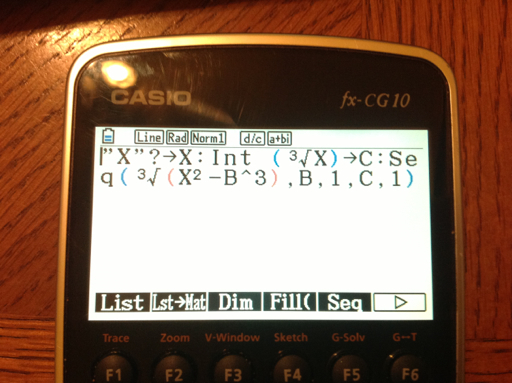The only solution that I found with X = 1 to 20 is:

(A=1, B=2, X=3). [A and B can be interchanged]
9 = 3² = 1³ + 2³ = 1 + 8

Stay safe and take care, Eddie. Thanks everyone.

This blog is property of Edward Shore. 2012

## Sunday, December 23, 2012

### More Equations Involving Natural Numbers

Greetings!

For the following let a, b, and n ∈ N. That is all variables are natural numbers (the counting numbers). Note that the set of natural numbers may or may not include 0. I will include 0 for this blog entry. This blog entry is all about trying to find solutions to equations - in an analytic manner.

a + b = a * b

If a = b, then:
a + a = a * a
2a = a^2

The only solution to this is a=2. (2*2 = 2^2 = 4)

Allowing for possibilities that a and b are different:
a + b = a * b
a + b - a * b = 0
a * (1 - b) = -b
a = -b/(1 - b) = b/(b - 1) = 1 + 1/(b - 1)

The only way that 1/(b - 1) ∈ N is when b = 2. Then a = 2.

The only solution to a + b = a * b is a = b = 2.

a^2 + b^2 = a * b

Let's say if a = b. Then:

a^2 + a^2 = a * a
2a^2 = a^2
a^2 = 0
a = 0

This implies that b=0. This works because 0^2 + 0^2 = 0 * 0 = 0.

What if we allow the possibility that a ≠ b?

a^2 + b^2 - a*b = 0
a^2 * (1 - b/a) + b^2 = 0

This lead me to believe that b is a multiple of a. Let b = a * n. Then:

a^2 * (1 - n) + a^2 * n^2 = 0

Assuming a ≠ 0,
(1 - n) + n^2 = 0

n = (1 ± i √3)/2.

Not good for searching for solutions such that a,b ∈ N.

In the general case:
a^2 + b^2 - a*b = 0
(1)(a^2) - (b)(a) + (b^2) = 0

Then:
a = (b ± √(b^2 - 4b^2))/2 = (b ± i b √3)/2 = b * (1 ± i √3)/2 which is not a natural number.

The only solution to a^2 + b^2 = a*b is a=b=0

a + b^2 = a * b

a + b^2 - a*b = 0
(1)(b^2) - (a)(b) + (a) = 0

b = (a ± √(a^2 - 4a))/2 = a/2 ± 1/2 * √(a^2 - 4a)

This implies that:
(1) a^2 - 4a must be a perfect square,
(2) a^2 - 4a must be even, and
(3) a must be even.

If a=2: √(2^2 - 4*2) = √(-4) = 2i. So a=2 is not a solution.

If a=4: √(4^2 - 4*4) = 0 which leads to b = 4/2 ± 0 = 2.
Then with a=4 and b=2:
4 + 2^2 = 4 * 2
8 = 8

A solution is found! Are there any more?

If a = 6, then a^2 - 4a = 12, not a perfect square.
If a = 8, then a^2 - 4a = 32, not a perfect square.
If a = 10, then a^2 - 4a = 60, not a perfect square.
If a = 12, then a^2 - 4a = 96, not a perfect square.
If a = 14, then a^2 - 4a = 140, not a perfect square.
If a = 16, then a^2 - 4a = 192, not a perfect square.

If a^2 - 4a is a prefect square, then a^2 - 4a = n^2. Then:
a^2 - 4a - n^2 = 0.
Then a = 2 ± √(4 + 2n^2). This is inconclusive.

One solution to a + b^2 = a * b is a=4 and b=2. There could be more.

a^2 + b^2 = 2 * a * b

Let's solve the equation in terms of b:
a^2 - 2 * a * b + b^2 = 0
(1)(b^2) - (2 * a)(b) + (a^2) = 0

And:
b = (2 * a ± √(4 * a^2 - 4 * a^2))/2 = (2 * a ± √0)/2 = a

This makes sense because when a=b, a^2 + a^2 = 2 * a * a = 2 * a^2.

Testing a few solutions:

a=3 and b=3:
3^2 + 3^2 = 18 and 2*3*3 = 18

a=6 and b=6:
6^2 + 6^2 = 72 and 2*6*6 = 72

The solution to a^2 + b^2 = 2*a*b is when a = b

Enjoy!

Eddie

This blog is property of Edward Shore. 2012

## Wednesday, December 19, 2012

### Equations without Whole Number Solutions - Fruitless Search?

Hi everyone! Hopefully you are fine today. Not long until 2013. And yes, I am 99.44% confident that the human race will still be on Earth be here come 12/22/2012.

x + x^2 = n^2

Let n,x ∈ N. N represents the natural numbers. Natural numbers are commonly referred to the whole numbers 1, 2, 3, etc. Some mathematicians include 0.

Are there any integer solutions to x + x^2 = n^2 with n,x ∈ N?

Our first instinct is most likely to go grab the nearest calculator or computer. Observe that:

(I). x + x^2 = n^2

(II). x^2 * (1/x + 1) = n^2

Taking the (principal) square root of both sides yields:

(III). x * √(1/x + 1) = n

In order for (III) to be true:
1. The quantity 1/x + 1 has to be an integer,
2. 1/x + 1 has to be a perfect square, and
3. x = 1/x + 1

The only natural number that allows condition 1 to be true is when x = 1.

Then 1/x + 1 = 2.

We know that 2 is not a perfect square (√2 ≈ 1.41421), so condition 2 fails. (II) does not fit because 1 * √2 = √2, leaving n = √2, not fitting the requirement that n,x ∈ N.

According to this analysis, there are no natural number solutions to x + x^2 = n^2.

x + x^2 + x^3 = n^q

Let n,x ∈ N. Suppose q = 2. Can we find solutions with these conditions?

x + x^2 + x^3 = n^2

x^2 * (1/x + 1 + x) = n^2

x * √(1/x + 1 + x) = n

Once again the only way 1/x + 1 + x is an integer is that when x = 1. However when x=1, 1/x + 1 + x = 3, and √3 ≈ 1.73205. And 1 * √3 = √3, n = √3, which is not a natural number.

There are no solutions (in the natural number set) for x + x^2 + x^3 = n^2.

Then x + x^2 + x^3 = n^3

x^3 * (1 + 1/x + 1/(x^2)) = n^3

x * ∛(1 + 1/x + 1/(x^2)) = n

Again, the only possible candidate is when x=1, but that leaves n = ∛3. No solutions in the natural number set.

Not all is "lost"...

Consider trying to find solutions to:

x + x^2 + x^3 + x^4 = n^2 where n,x ∈ N.

Then x * √(1/x + 1 + x + x^2) = n.

If x = 1, then √(1/x + 1 + x + x^2) = √4 = 2, and n = 1 * 2 = 2. Success! I believe x=1 and n=2 is the only solution to this equation with these conditions imposed.

Happy Holidays everyone and see you next time!

Eddie

This blog is property of Edward Shore. 2012

## Sunday, December 16, 2012

### Adding Numbers and their Reverses

Please take a look at the Fun With Num3ers blog by Benjamin Vitale (Twitter: @BenVitale). This is a fun blog working with number theory. It is also an inspiration for me to blog. Thanks, Ben!

Eddie

On to today's blog entry!

Two Digit Numbers

Let AB represent a two digit number, where A and B each represent a digit 0 through 9 (excluding 00). My original goal was to find a number AB such that AB + BA = n where n contains both digits A and B. I was unsuccessful.

However, I did see something curious:

11 + 11 = 22
12 + 21 = 33
13 + 31 = 44
14 + 41 = 55
15 + 51 = 66
16 + 61 = 77
17 + 71 = 88
18 + 81 = 99
19 + 91 = 110
10 + 01 = 10 + 1 = 11

All the sums for this group are multiples of 11.

If I go on...
21 + 12 = 33
22 + 22 = 44
23 + 32 = 55
24 + 42 = 66
25 + 52 = 77
....

Once again, multiples of 11. Can we show that this is true for all sums AB + BA?

Let a represent the digit A. Similarly, let b represent the digit B. Then:

AB = (10a + b) and BA = (10b + a)

Then the sum AB + BA = (10a + b) + (10b + a) = 11a + 11b = 11(a + b)

Three Digit Numbers

Let's consider the number ABC. Rotate ABC one digit and add. Repeat. We get the sum ABC + BCA + CAB = n.

Let a, b, and c represent the digits A, B, and C, respectively. Then ABC = 100a + 10b + c. We can construct two similar sums for BCA and CAB.

Then

ABC + BCA + CAB
= (100a + 10b + c) + (100b + 10c + a) + (100c + 10a + b)
= 111a + 111b + 111c
= 111(a + b + c)

The sum is ends up a multiple of 111, but not necessarily of 11. (111 is not a multiple of 11). Examples?

Example 1:
425 + 254 + 542 = 1221
1221/111 = 11
1221/11 = 111

Example 2:
963 + 639 + 396 = 1998
1998/111 = 18
1998/11 = 181 6/11
1998 is not a multiple of 11

Can we find such triples ABC, BCA, and CAB such that the sum is divisible by both 11 and 111? Hint: the least common multiple of 11 and 111 is 1,221. I have found 31 such triplets (including the trivial 0,0,0).

If you want to find the triples, have fun! I'll leave you with a few examples:

29 + 902 + 290 = 1221. (029, 902, 290)
137 + 713 + 371 = 1221. (137, 713, 371)
598 + 859 + 985 = 2442. (598, 859, 985)
254 + 425 + 542 = 1221. (254, 425, 542)

Take care and be safe!

Eddie

This blog is property of Edward Shore. 2012

## Saturday, December 15, 2012

### Trigonometric Functions with Random Number Arguments

Sine

Let R be a random number such that 0 ≤ R ≤ 1.

Then 0 ≤ R x ≤ x.

Since sin(0) = 0, we can simply conclude that 0 ≤ sin(R x) ≤ sin x, right?

However, we know that -1 ≤ sin x ≤ 1. Does this fact invalidate the claim that 0 ≤ sin(R x) ≤ sin x? Let's take a look at sin x vs sin(R x) graphically.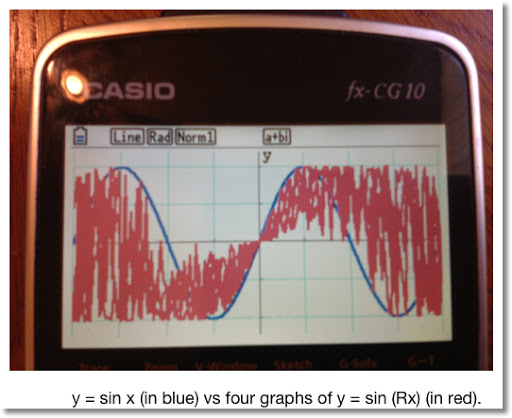From the graph, we can clearly see that 0 ≤ sin (Rx) ≤ sin x. Let's take a look at the region x ∈ [0, 2π].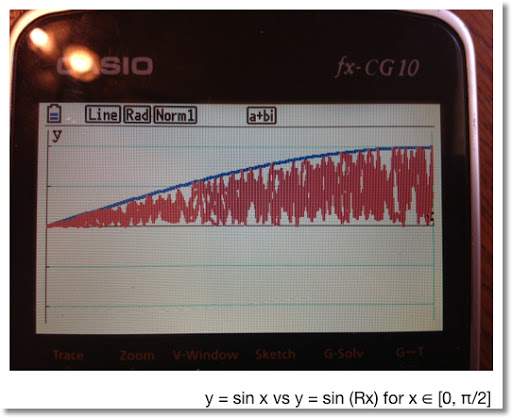In the region x ∈ [0, π/2], we can clearly see that 0 ≤ sin (Rx) ≤ sin x.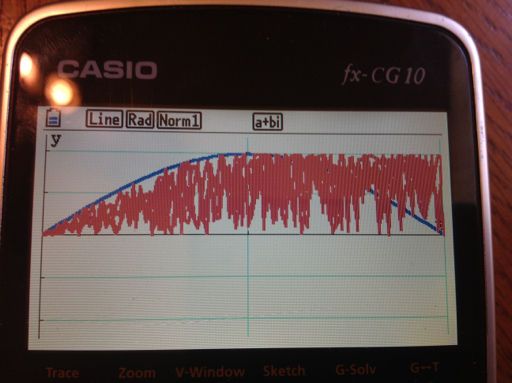As we explore the next region x ∈ [0, π], we still get positive values for sin (Rx). However, since R takes the value between 0 and 1, we get values 0 ≤ sin (Rx) ≤ 1.

Case: if R = 0.5 and x = π, sin(Rx) = 1.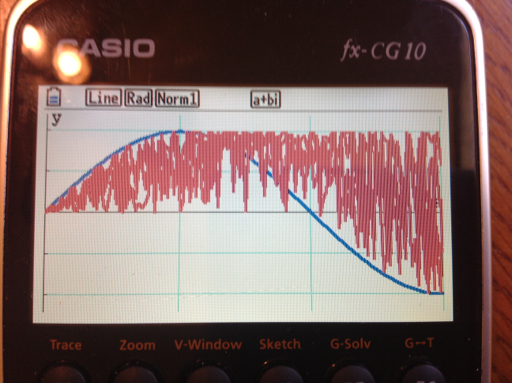When x ∈ [π/2, 3 π/2], the value of sin x declines below 0. However, since R is random, sin(Rx) takes the values sin x to 1.

Note that when x = 3π/2, sin(x) = -1.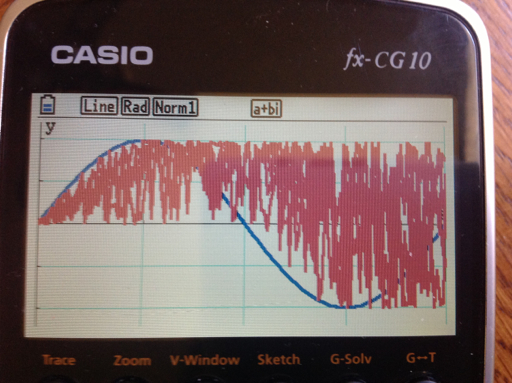At the final sub interval x ∈ [3π/2, 2 π], sin (Rx) takes on all values in the interval [-1, 1].

To summarize:

When 0 ≤ x ≤ π/2, 0 ≤ sin (Rx) ≤ sin x.
When π/2 ≤ x ≤ π, 0 ≤ sin (Rx) ≤ 1.
When π ≤ x ≤ 3π/2, sin x ≤ sin (Rx) ≤ 1.
When 3π/2 ≤ x ≤ 1, -1 ≤ sin (Rx) ≤ 1.

Interesting that we can't just say 0 ≤ sin (Rx) ≤ sin x for all x.

Cosine

We can make the similar argument that even though:

0 ≤ R ≤ 1, 0 ≤ R x ≤ x, and cos(0) = 1,

The statement 1 ≤ cos(Rx) ≤ cos x is not true for all x. Looking the graph cos x vs cos (Rx):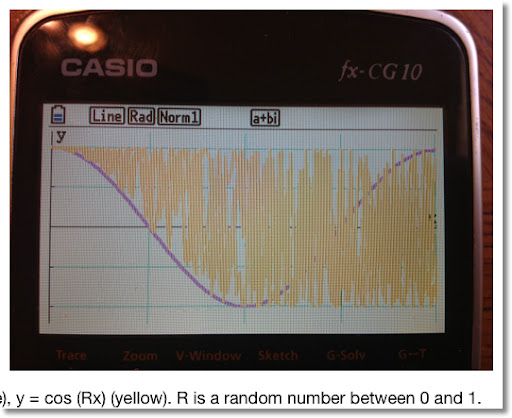Looking at the sub-intervals of [0, 2π]:

When 0 ≤ x ≤ π/2, cos x ≤ cos (Rx) ≤ 1.
When π/2 ≤ x ≤ π, π/2 ≤ cos (Rx) ≤ 1.
When π ≤ x ≤ 3π/2, -1 ≤ cos (Rx) ≤ 1.
When 3 π/2 ≤ x ≤ π, -1 ≤ cos (Rx) ≤ 1.

When random numbers are involved with functions, interesting things happen.

sin (Rx) cos (Rx) vs. 1/2 sin (2Rx)

Using the trig identity:

(sin x) * (cos x) = 1/2 * sin(2x)

Let R represent a random number function which returns a random number between 0 and 1. On a calculator, each time the random number function is called, a different random number is returned.

I will leave this as an open question: what happens at each interval [0, π/2], [π/2, π], [π, 3π/2], and [3π/2, 2 π]? I'll end this blog entry with a graphing comparison.

Note the range of sin(Rx) cos(Rx) is [-1, 1].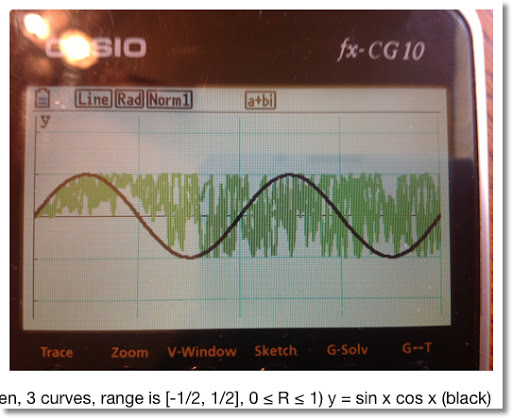Stay safe everyone! Eddie

This blog is property of Edward Shore. 2012

## Friday, December 14, 2012

### Random Numbers and Polynomials

Random Numbers and Polynomials

Let R be a random number such that 0 ≤ R ≤ 1. Then the inequality by x four times. Then:

0 ≤ R ≤ 1

0 ≤ R x ≤ x

0 ≤ R x^2 ≤ x^2

0 ≤ R x^3 ≤ x^3

0 ≤ R x^4 ≤ x^4

And for any integer n,

0 ≤ R x^n ≤ x^n

If we try to graph R x on a graphing calculator, we can imagine that the calculator generates a random R between 0 and 1 for each x. Graphing four y = R x on top of each other produces a result like this:Here are the graphs for y = R x^2, and y = R x^3, respectively.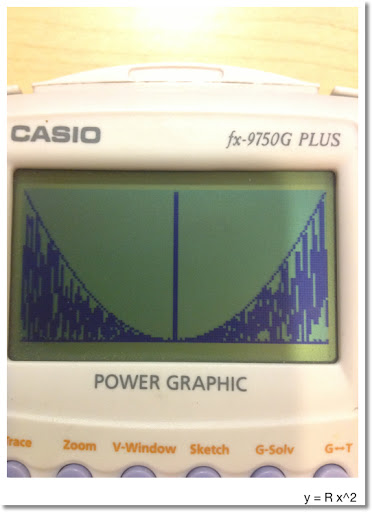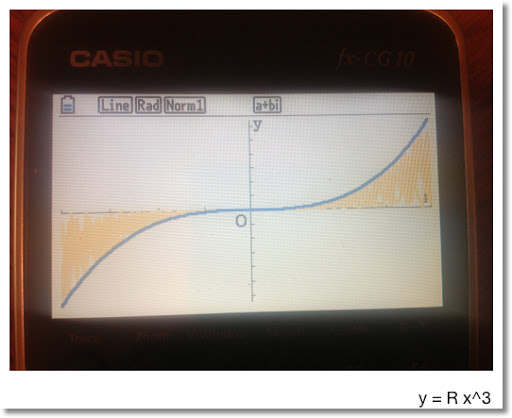Note that:

For odd n and x ≥ 0:

0 ≤ R x^n ≤ x^n

For odd n and x < 0:

0 ≥ R x^n ≥ x^n

For even n:

0 ≤ R x^n ≤ x^n

An Interesting Thing When Building Polynomials

Consider the function: y = Rx^2 + Rx + R

We can look at this two ways:

1. Treat R as a constant random number. Then y = R(x^2 + x + 1)

2. Treat each R as a separate random number, 0 ≤ R ≤ 1. In fact, when you put the function y = Rand# x^2 + Rand# x + Rand#, the calculator treats Rand# as a separate command.

Let's look at a comparison, where y = Rand# (x^2 + x + 1) is in red and y = Rand# x^2 + Rand# x + Rand# is in blue. Recall that Rand# is a random number function.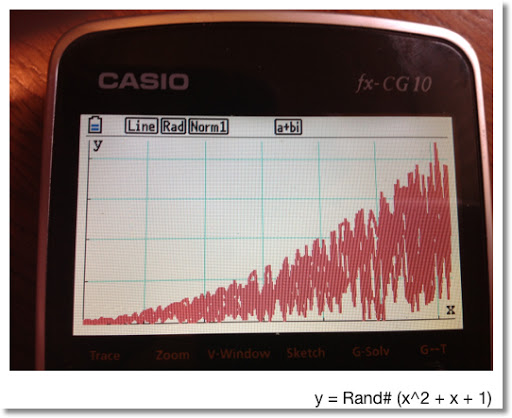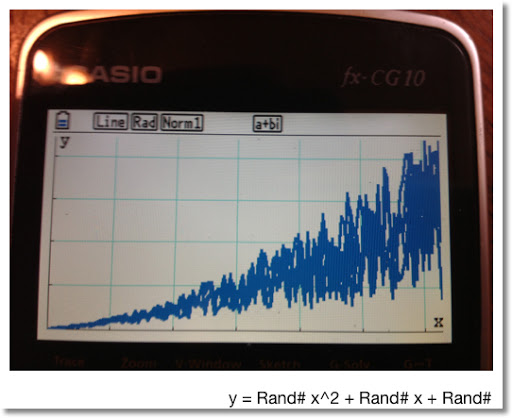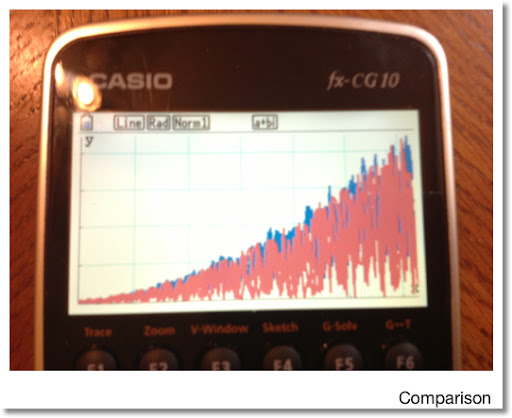That is it. Stay safe everyone, it is a crazy world! Eddie

This blog is property of Edward Shore. 2012

## Wednesday, December 12, 2012

### Happy 12/12/12 Day!

Celebrate the number 12 today! Have a great day everyone. Eddie

## Wednesday, December 5, 2012

### Numeric CAS - Part 12: Roots of a Complex Numbers

Complex Roots

Goal: Find all the complex roots of the complex number z.

This the solution to the equation:

x^n - z = 0

Where z = a + bi

Using de Moivre's Theorem:

Let r = abs(z) = √(x^2 + y^2) and
θ = arg(z) = arctan(y/x) where -π/2 ≤ θ ≤ π/2,

Then z^(1/n) = r^(1/n) * cos((2 k π + θ)/n) + i * r^(1/n) * sin((2 k π + θ)/n)
Where k = 0, 1, ... , n-1

Example:
Find the three roots of 1. ( ∛1 ) (n=3)

Roots: ≈ 1, -0.5 + 0.8660i, -0.5 - 0.8660i

Find the four roots of 2 + 7i. (n=4)

Roots: ≈ 1.5576 + 0.5216i, -0.5216 + 1.5576i, -1.5576 - 0.5216i, 0.5216 - 1.5576i

Casio Prizm:

CROOTS
Complex Roots
11/23/2012

(Prizm, fx-9860g, fx-9750gii - 92 bytes)
a+bi
"a+bi"? → Z
"ROOT"? → N
For 0 → K To N-1
N x√ (Abs Z) × e^( i (Arg Z + 2Kπ) ÷ N) ◢
Next

Alternative (done on CFX-9850G, older Casios - 94 bytes)
"A+Bi"? → Z
"ROOT"? → N
For 0 → K To N-1
N x√ (Abs Z) × ( cos ((Arg Z + 2Kπ) ÷ N) + i sin ((Arg Z + 2Kπ) ÷ N)) ◢
Next

TI-84+:

CROOTS
Complex Roots (TI-83+/TI-84+)
11/23/2012
76 Bytes

a+bi
Input "a+bi:", Z
Input "ROOT:", N
For(K,0,N-1)
Pause N x√ (abs(Z)) * e^(i(angle(Z)+2Kπ )/N)
End

HP 39gii:

PROGRAM CROOTS
All the N complex roots of Z
11/23/2012

Input: CROOTS(Z,N)

EXPORT CROOTS(Z,N)
BEGIN
LOCAL L3,K;
0 → HAngle; // set calculator to Radians mode
{ } → L3;
FOR K FROM 0 TO N-1 DO
CONCAT(L3, {N NTHROOT (ABS(Z)) * e^( i ( ARG(Z) + 2 * K * π )/N) → L3;
END;
RETURN L3;
END;

This concludes the Numeric CAS series... For now.

Until next time, Eddie

This blog is property of Edward Shore. 2012

### Numeric CAS - Part 11: Eigenvalues of 3 x 3 Matrices

Eigenvalues of 3 x 3 Matrices

Many graphing calculators that do not have CAS (computer algebraic systems) do not have eigenvalues and eigenvector functions.

The programs for the Casio Prizm and TI-84+ gives eigenvalues of 3 x 3 matrices.

For the HP 39gii has the functions EIGENVAL for eigenvalues.

Example:
A = [[2, -2, 3][-1, 0, 1][6, -3, 3]]

Eigenvalues:
≈ 0.2465, -1.8447, 6.5982

Casio Prizm:

EIGEN3
Eigenvalues of a 3 × 3 Matrix
Circa 2011 - 244 bytes

a+bi
"3 × 3 Matrix"? → Mat A
Mat A[1,1] + Mat A[2,2] + Mat A[3,3] → T
(Mat A)² → Mat B
Mat B[1,1] + Mat B[2,2] + Mat B[3,3] → U
Solve(-X^3 + X^2 × T + X × 1/2 × (U-T^2) + Det Mat A,0)→ R ◢
-R^2 + R × T - T^2 ÷ 2 + U ÷ 2 → A
-R + T → B
1/2 × (B - √(4A + B^2)) → E ◢
1/2 × (B + √(4A + B^2)) → F ◢
"STORED IN R, E, F"

TI-84+:

EIGEN3
Eigenvalues of a 3 × 3 matrix - 193 bytes
12/3/12

a+bi
Input "3 X 3 MATRIX:", [A]
[A](1,1) + [A](2,2) + [A](3,3) → T
[A]² → [B]
[B](1,1) + [B](2,2) + [B](3,3) → U
solve(-X³ + X² T + .5X(U-T²) + det([A]), X, 0) → R
Pause R
-R² + RT - T²/2 + U/2 → A
-R + T → B
.5(B - √(4A+B²)) → E
.5(B + √(4A+B²)) → F
Pause E
Pause F

This blog is property of Edward Shore. 2012

### Numeric CAS Part 10 - Eigenvalues of 2 x 2 Matrices

Eigenvalues of 2 x 2 Matrices

Many graphing calculators that do not have CAS (computer algebraic systems) do not have eigenvalues and eigenvector functions.

The program for the Casio Prizm gives eigenvalues. The TI-84+ program gives both eigenvalues and eigenvectors.

For the HP 39gii has the functions EIGENVAL and EIGENVV for eigenvalues and eigenvectors, respectively.

Example:
A = [[2, 7][-3, 9]]

Eigenvalues: ≈ 5.5 ± 2.9580i

Casio Prizm:

Eigenvalues of a 2 × 2 Matrix
EIGEN2 - 136 Bytes

a+bi
"2 × 2 MATRIX:"? → Mat A
Mat A[1,1] + Mat A[2,2] → T
Det Mat A→ D
(T+√(T^2-4D))÷2 → R ◢
(T-√(T^2-4D))÷2 → S ◢
"λS STORED IN R,S"

TI-84+:

EIGEN2
Eigenvalues of 2 × 2 matrices - Approximately 280 bytes
Bonus! A pair of eigenvectors are given
5/15/2011

a+bi
Input "2 X 2 MATRIX:", [J]
[J](1,1) + [J](2,2) → T
det([J]) → D
(T + √(T^2 - 4D))/2 → U
(T - √(T^2 - 4D))/2 → V
Disp "EIGENVAL. U"
Pause U
(U - [J](1,1)) / [J](1,2) → A
√(1 + A^2)⁻¹ * [[A]] → [H]
Disp "EIGENVEC. [H]"
Pause [H]
(V - [J](1,1)) / [J](1,2) → B
√(1 + B^2)⁻¹ * [[B]] → [I]
Disp "EIGENVAL. V"
Pause V
Disp "EIGENVECT. [I]"
Pause [I]

This blog is property of Edward Shore. 2012

### Numeric CAS - Part 9: Indefinite Integrals of Polynomial

Indefinite Integral of a Polynomial

Goal: Determine the coefficients of the indefinite integral of the polynomial p(X) where

p(X) = a_n * x^n + a_(n-1) * x^(n-1) + ... + a1 * x + a0

and

∫ p(X) dX = a_n * x^(n+1) ÷ (n+1) + a_(n-1) * x^n ÷ n + ... + a0 * x + c

Where c is a constant. This constant is determined by the initial condition (x_0, p_0). The programs assume that c = 0, which is common in computer algebraic systems.

Where a_n, a_(n-1), ... , a1, a0 are stored in lists. The coefficients are listed in order of descending powers of x. Use zeros as placeholders.

Example:

∫ -2x^2 + 4x - 6 dx = -2/3 * x^3 + 2x^2 - 6x
Input List: {-2, 4, -6}
Output List: {-2/3, 2, -6, 0} or
{-0.666666667, 2, -6, 0}

∫ x^4 + 3x^2 - x dx = 1/5 * x^5 + x^3 - 1/2 * x^2
Input List: {1, 0, 3, -1, 0}
Output List: {1/5, 0, 1, -1/2, 0, 0} or
{0.2, 0, 1, -0.5, 0, 0}

For all three programs, the input is List 1, the output is List 2.

Casio Prizm:

POLYINT
Indefinite Polynomial Integral - 140 bytes

"∫ (P(X)) dX"
"{AnX^n,...,A0}"
"LIST="?→List 1
List 1 → List 2
For 1→K To Dim List 1
List 2[K] ÷ (Dim List 2 + 1 - K) → List 2[K]
Next
Augment(List 2,{0}) → List 2
List 2

TI-84+:

POLYINT
Polynomial Indefinite Integral - 117 bytes

: Disp "fnInt(P(X)) DX", "{AnX^n,...,A0}"
: Input "LIST:", L1
: L1 → L2
: For(K,1,dim(L2))
: L2(K)/(dim(L2)-K+1) → L2(K)
: End
: augment(L2,{0}) → L2
: Pause L2

HP 39gii:

Polynomial Integral
POLYINT()
Same input as above

EXPORT POLYINT()
BEGIN
LOCAL K,S;
EDITLIST(L1);
SIZE(L1)→S;
{ } → L2;
FOR K FROM 1 TO S DO
CONCAT(L2, {(S-K+1)⁻¹ * L1(K)}) → L2;
END;
CONCAT(L2,{0})→ L2;
RETURN L2;
END;

This blog is property of Edward Shore. 2012

### Numeric CAS - Part 8: Polynomial Derivative

Derivative of a Polynomial

Goal: Determine the coefficients of the derivative of the polynomial p(X) where

p(X) = a_n * x^n + a_(n-1) * x^(n-1) + ... + a1 * x + a0

and

d/dX p(X) = a_n * n * x^(n-1) + a_(n-1) * (n-1) * x^(n-2) + ... + a1

Where a_n, a_(n-1), ... , a1, a0 are stored in lists. The coefficients are listed in order of descending powers of x. Use zeros as placeholders.

Example:

d/dx -2x^2 + 4x - 6 = -4x + 4
Input List: {-2, 4, -6}
Output List: {-4, 4}

d/dx x^4 + 3x^2 - x = 4x^3 + 6x - 1
Input List: {1, 0, 3, -1, 0}
Output List: {4, 0, 6, -1}

For all three programs, the input is List 1, the output is List 2.

Casio Prizm:

POLYDX
Polynomial Derivative - 132 bytes

"d/dX P(X)"
"{AnX^n,...,A0}"
"LIST:"? → List 1
Dim List 1-1 → Dim List 2
For 1→K To Dim List 2
(Dim List 1-K) × List 1[K] → List 2[K]
Next
List 2

TI-84+:

POLYDX
Polynomial Derivative - 118 bytes

: Disp "D/DX P(X)", "{AnX^n,...,A0}"
: Input "LIST:",L1
: L1->L2
: dim(L2)-1->dim(L2)
: For(K,1,dim(L2))
: (dim(L2)-(K-1))*L2(K)->L2(K)
: End
: Pause L2

HP 39gii:

Polynomial Derivative
11/23/2012 - edited 12/2/2012

POLYDX()

EXPORT POLYDX()
BEGIN
LOCAL K,S;
EDITLIST(L1);
SIZE(L1)→S;
{ } → L2;
FOR K FROM 1 TO S-1 DO
CONCAT(L2, {(S-K)*L1(K)}) → L2;
END;
RETURN L2;
END;

This blog is property of Edward Shore. 2012

## Monday, December 3, 2012

### Numeric CAS - Part 7: Quadratic Equation

Ax² + Bx + C = 0

Where x = (-B ± √(B² - 4AC)) / (2A)

This is just the popular quadratic formula, except this set of programs allow for complex A, B, and C. (Unlike the Solver applications).

Example:
A = 2 - i
B = -6
C = 14i

x1 ≈ -0.9873 + 1.551i
x2 ≈ 3.3873 - 0.3509i

Casio Prizm:

Quadratic Equation - Complex Coefficients Allowed
11/23/2012 - 124 bytes

a+bi
"AX²+BX+C=0"
"A"? → A
"B?"→ B
"C?"→ C
√(B²-4AC) → X
(-B - X) ÷ (2A)→ R
(-B + X) ÷ (2A) → S
"X=R Or X=S"
R ◢
S

TI-84+:

Quadratic Formula - Allows for Complex Coefficients
Ax^2 + Bx + C = 0

a+bi
Disp "AX²+BX+C"
Prompt A,B,C
√(B²-4AC) → X
(-B - X)/(2A) → R
(-B + X)/(2A) → S
Disp "X=R or X=S"
Pause R
Pause S

HP 39gii:

Use the POLYROOT command. POLYROOT([A,B,C])

The vector can represent any polynomial.

This blog is property of Edward Shore. 2012

### Numeric CAS Part 6: Euclid Algorithm to find GCD (Greatest Common Divisor)

Euclid Algorithm

Goal: To find the greatest common divisor of two integers U and V.

The Algorithm in Brief:

Let U ≥ V. Then U = A * V + R where A is the quotient and R is the remainder. If R≠0, then V becomes the new U, and R becomes the new V. The process repeats until you R=0. When that happens, V_last = GCD(U,V).

The program allows for you two enter integers in any order. Each program uses the home screen to display the steps, but the HP 39gii displays all the steps on one screen.

Example: gcd(166, 78)

166 = 2 * 78 + 10
78 = 7 * 10 + 8
10 = 1 * 8 + 2
8 = 4 * 2 + 0
GCD = 2

gcd(169,39)

169 = 4 * 39 + 13
39 = 3 * 13 + 0
GCD = 13

Casio Prizm:

EUCLID
GCD Program - 12/3/12
212 bytes

"X"?→ X
"Y"?→ Y
If X ≥ Y
Then X → M
Y → N
Else X → N
Y → M
IfEnd
Lbl 1
Int(M ÷ N) → A
M - A × N → R
ClrText
Locate 1,2,M
Locate 1,3,"="
Locate 2,3,A
Locate 13,3,"×"
Locate 14,3,N
Locate 1,4,"+"
Locate 2,4,R ◢
If R=0
Then Goto 2
Else N → M
R → N
Goto 1
IfEnd
Lbl 2
Locate 1,6,"GCD="
Locate 6,6,N

TI-84+:

GCD by Euclidian Algorithm
11/25/2012 - 160 bytes

Program EUCLID

Prompt X,Y
min(X,Y) → N
max(X,Y) → M
Lbl 1
iPart(M/N) → A
M - A * N → R
ClrHome
Output(2,1,M)
Output(3,1,"=")
Output(3,2,A)
Output(3,8,"*")
Output(3,9,N)
Output(4,1,"+")
Output(4,2,R)
Pause
If R=0
Then
Goto 2
Else
N → M
R → N
Goto 1
End
Lbl 2
ClrHome
Disp "GCD=", N

HP 39gii:

Program EUCLID
Finding the GCD by the Euclid algorithm
10/16/2012

Input: EUCLID(X,Y)

EXPORT EUCLID(X,Y)
BEGIN
LOCAL M,N,R,A;
MAX(X,Y)→ N;
MIN(X,Y)→M;
PRINT();
REPEAT
INT(N/M)→ A;
N - A*M→ R;
PRINT(A + "*" + M + "+" + R);
WAIT(1);
M→ N;
R→ M;
UNTIL R==0;
RETURN N;
END;

This blog is property of Edward Shore. 2012

## Sunday, December 2, 2012

### Numeric CAS - Part 5: Polynomial Evaluation

Polynomial Evaluation

Goal: Evaluation of the polynomial p(x) at x = a. This gives an alternative to typing out the polynomial itself.

Enter the coefficients of the polynomial as a list, in descending order from highest power to constant. Use zeros as place-holders.

Example:
Let p(x) = 2x^4 + 2x^2 - 4x + 1 at x = -1.5

List Input: {2, 0, 2, -4, 1}
Enter -1.5 when the program asks for X.

Result: p(-1.5) = 21.625

Casio Prizm:

POLYEVAL
Poly Evaluation
11/23/2012
(140 bytes)

Example: p(x) = x^3 + 2x^2 + 5x + 9, p(-2) = -1

Program:
"{AnX^n,...,A0}"
"P(X)"? → List 1
"X"? → X
X = 0 ⇒ Goto 1
0 → S
For 1 → K To Dim List 1
S + List 1[K] × X^(Dim List 1 - K) → S
Next
S
Stop
Lbl 1
List 1[Dim List 1]→ S

Sum is stored in S, 0^0 is not allowed.

TI-84+:

POLYEVAL
Polynomial Evaluation
11/23/2012 - 124 bytes

Example: p(x) = 1.425x^3 - 2.89x^2 + 0.23x + 4.4546
p(1.002) = 3.217055551

For the character n: VARS, 5, 1

Disp "{AnX^n,...,A0}"
Input "P(X):", L1
Input "X:", X
If X=0
Goto 1
0→ S
For(K,1,dim(L1))
S + L1(K) * X^(dim(L1) - K) → S
End
Pause S
Stop
Lbl 1
L1(dim(L1)) → S
Pause S

HP 39gii:

There is no program. Instead, use the POLYEVAL command.

POLYEVAL(vector, value)

The vector contains the coefficients of the polynomial.

This blog is property of Edward Shore. 2012

### Numeric CAS - Part 4: Polynomial Multiplication

Polynomial Multiplication

Goal: Multiply two polynomials: p1(x) * p2(x) = p3(x)

Inputs: Coefficients of p1(x) and p2(x) as List 1 and List 2, respectively

Output: Coefficients of p3(x) as List 3.

The lists are in order from highest order to constant. Zeros are used as place holders.

Example: (x^3 + 2x - 1) * (-2x^2 + x - 4) = -2x^5 + x^4 - 8x^3 + 4x^2 - 9x + 4

Input:
List 1: {1, 0, 2, -1}
List 2: {-2, 1, -4}

Output:
List 3: {-2, 1, -8, 4, -9, 4}

Casio Prizm:

POLYMULT
Multiplication of Two Polynomials
11/21/2012 - 176 bytes

"{AnX^n,...,A0}"
"P1(X)"? → List 1
"P2(X)"? → List 2
Dim List 1 + Dim List 2 - 1 → Dim List 3
For 1 → K To Dim List 1
List 1[K] × List 2 → List 4
For 1 → J To Dim List 4
List 3[J + K - 1] + List 4[J] → List 3[J + K - 1]
Next
Next
List 3

Example:
(x^2 + 3x - 1)(x^2 - 4) = x^4 + 3x^3 - 5x^2 - 12x + 4
List 1: {1,3,-1}
List 2: {1,0,-4}
Result List 3: {1,3,-5,-12,4}

TI-84+:

POLYMULT
Multiplication of two Polynomials
{AnX^n,...,A0}
11/21/2012 - 154 bytes

To get the lower case "n" character: VARS, 5, 1

Disp "{AnX^n,...,A0}"
Input "P1(X)=", L1
Input "P2(X)=", L2
dim(L1) + dim(L2) - 1 → dim(L3)
Fill(0,L3)
For(K, 1, dim(L1))
L1(K) * L2 → L4
For(J, 1, dim(L4))
L3(J + K - 1) + L4(J) → L3(J + K - 1)
End
End
Pause L3

HP 39gii:

Polynomial Multiplication (FULL)
12/2/2012

EXPORT POLYMULT()
BEGIN
LOCAL S,K,J;
EDITLIST(L1);
EDITLIST(L2);
SIZE(L1)+SIZE(L2)-1 → S;
MAKELIST(0,X,1,S,1) → L3;
FOR K FROM 1 TO SIZE(L1) DO
L1(K)*L2 → L4;
FOR J FROM 1 TO SIZE(L4) DO
L3(J+K-1) + L4(J) → L3(J+K-1);
END;
END;
RETURN L3;
END;

This blog is property of Edward Shore. 2012

### Numeric CAS - Part 3: Synthetic Division

Synthetic Division

Goal: Divide the polynomial p(x) = a_n x^n + a_(n-1) x^(n-1) + ... + a_0 by (x - r).

p(x) ÷ (x - r) = q_n x^n + q_(n-1) x^(n-1) + ... + q_0 with remainder E

Input: A list of coefficients {a_n, a_(n-1), a_(n-2), ... , a_0} (List 1)

Output: Resulting coefficients {q_n, q_(n-1), ... , q_0, E} (List 2)

Example: (2x^3 + x - 3) ÷ (x - 3) = 2x^2 + 6x + 19 + 54 ÷ (x - 3)

List 1: {2, 0, 1, -3}, R = 3
List 2: {2, 6, 19, 54}

Casio Prizm

POLYSYN
Synthetic Division - 160 bytes

"P(X) ÷ (X-R)"
"{AnX^n,...,A0}"
"LIST:"?→List 1
"R"?→R
List 1 → List 2
For 1→K To Dim List 1 - 1
R × List 2[K] + List 1[K+1] → List 2[K+1]
Next
"LAST TERM = REMAINDER" ◢
List 2

TI-84+

POLYSYN
Synthetic Division - 143 bytes

: Disp "P(X)/(X-R)"
: Disp "{AnX^n,...,A0}"
: Input "LIST:", L1
: Input "R:", R
: L1->L2
: For(K,1,dim(L1)-1)
: R*L2(K)+L1(K+1)->L2(K+1)
: End
: Disp "LAST TERM=", "REMAINDER"
: Pause L2

HP 39gii

POLYSYN
Synthetic Division
11/23/2012

L1 and A are prompted. The resulting is a list of coefficients, with the last term the remainder

Example: (21x^2 + 42x + 144)/(x - 12) = 21x + 294 + 3672/(x-12)
Output List: {21, 294, 3672}

EXPORT POLYSYN()
BEGIN
LOCAL K,S,T;
EDITLIST(L1);
INPUT(R,"P(X)/(X-R)");
L1 → L2;
SIZE(L1)→ S;
S-1 → T;
FOR K FROM 1 TO T DO
R * L2(K) + L1(K+1) → L2(K+1);
END;
MSGBOX("LAST TERM=REMAINDER");
RETURN L2;
END;

This blog is property of Edward Shore. 2012

## Saturday, December 1, 2012

### Numeric CAS Part 2: Binomial Expansion

Binomial Expansion

Goal: To find the coefficients of when (ax + by)^n is expanded. The variables a and b are numeric.

(ax + by)^n = Σ (n nCr k *(ax)^(n-k) * (by)^k for k = 0 to n).

Example: (3x - 2y)^3 = 27 x^3 - 54 x^2 y + 36 x y^2 - 8 y^3

For the Casio Prizm and TI-84+, each coefficient is given in order. They are also stored in List 6. For the HP 39gii, a string is built representing the expanded binomial.

The list in the above example is {27, -54, 36, -8}Casio Prizm:

POLYBINE
Binary Expansion
216 bytes

Lbl 6
"(AX+BY)^N"
"A"?→ A
"B"?→ B
"N"?→ N
If N≤0 Or Frac N≠0
Then
"N NOT AN INTEGER > 0" ◢
Goto 6
IfEnd
For 0 → K To N
ClrText
N nCr K × A ^ (N - K) × B ^ K → C
Locate 1,2,C
Locate 1,3,"×X^"
Locate 4,3,N-K
Locate 7,3,"×Y^"
Locate 10,3,K ◢
C→ List 1[K+1]
Next

Thanks to Ryan Maziarz for pointing out an extra quotation mark I had on line 3  (originally "A"?"A).  2/6/2013

Note:  The program will show the coefficients of the expansion one at a time.  You can view the entire list of the coefficients in List 1.

TI-84+:

POLYBINE
Binomial Expansion
177 bytes

: Lbl 1
: Disp "(AX+BY)^N"
: Prompt A,B,N
: If N≤0 or fPart(N)≠0
: Then
: Disp "NEED INTEGER>0"
: Pause
: Goto 1
: End
: N+1->dim(L6)
: For(K,0,N)
: N nCr K*A^(N-K)*B^K->C
: ClrHome
: Output(2,1,C)
: Output(3,1,"*X^")
: Output(3,4,N-K)
: Output(3,7,"*Y^")
: Output(3,10,K)
: Pause
: C->L6(K+1)
: End

Note:  The program will show the coefficients of the expansion one at a time.  You can view the entire list of the coefficients in L6.

HP 39gii:

POLYBINE
11/23/2012
Polynomial Binomial Expansion
Expand (ax + by)^n

The results are returned in a string. Note, if N is not a positive integer, it is converted into one.

Input: POLYBINE(A,B,C)

EXPORT POLYBINE(A,B,C)
BEGIN
LOCAL S1,S2,K;
ABS(INT(N)) → N;
"" → S1;
FOR K FROM 0 TO N DO
string(COMB(N,K)*A^(N-K)*B^K) → S2;
S1 + "+" + S2 + "*X^" + string(N-K) + "*Y^" + string(K) → S1;
END;
dim(S1) → K;
right(S1,K-1) → S1;
RETURN S1;
END;

This blog is property of Edward Shore. 2012

### Numeric CAS Part 1: Simplifying Square Roots

Simplifying Square Roots

Goal: Simplify square roots of integers. For example, √180 = 6 √5, √80 = 4 √5

General Algorithm: Start with integer N and C=1. Starting with k=2, divide N by k². If N divides k² evenly, N is adjusted, C is multiplied by k, and the division is test is repeated. If N does not not divide k², k increases by 1 and the division test repeats. The division tests repeat until k² > N.

The program for the Casio Prizm, TI-84+, and HP 39gii are presented below:

Casio Prizm:

SQFACTOR
11/19/2012
Simplifies √N where N is an integer (i.e. √180 = 6 √5, √364 = 2 √91)
156 bytes

"√N="? → N
N → M
1 → C
2 → K
Do
Lbl 1
If Frac(N ÷ K²)=0
Then
N ÷ K² → N
C × K → C
Goto 1
IfEnd
K + 1 → K
LpWhile K²ClrText
Locate 1,1,"√"
Locate 2,1,M
Locate 1,3,C
Locate 10,3,"×√"
Locate 12,3,N

TI-84+:

SQFACTOR
Square Root Simplification
(I.E. √180 = 6 √5 , √364 = 2 √91)
11/19/2012
133 bytes

Input "√(", N
N → M
1 → C
2 → K
Lbl 0
If fPart(N/K²)=0
Goto 1
1 + K → K
If K² < N
Goto 0
ClrHome
Output(1,1,"√(")
Output(1,3,M)
Output(3,1,C)
Output(3,7,"√(")
Output(3,9,N)
Stop
Lbl 1
N/K² → N
C*K → C
Goto 0

HP 39gii:

SQFACTOR
11/23/2012
Simplifies √N where N is an integer (i.e. √180 = 6 √5, √364 = 2 √91)

Input: SQFACTOR(N)

EXPORT SQFACTOR(N)
BEGIN
LOCAL C,K;
1 → C;
2 → K;
WHILE K² < N DO
WHILE FRAC(N/K²) == 0 DO
N/K² → N;
C*K→ C;
END;
K+1→K;
END;
RETURN string(C)+"√"+string(N);
END;

This blog is property of Edward Shore. 2012

### Numeric CAS Project - an Introduction

It's coming! The Numeric CAS project is a set of programs designed to give common graphing calculators a set of some common computer algebraic system functions and advanced graphing calculators.

The calculators that I will be working with in this series are:

* Texas Instruments TI-84 Plus
* Casio Prizm fx-CG 10 (should work on most Casio graphing calculators)
* Hewlett Packard 39gii

Everything is this series is done by using the calculator's native languages. No hacking is required. The programs use the numeric features of the calculator.

Often, lists will be used to represent coefficients of polynomials. The power of coefficients are in descending order. For example, to represent the polynomial x^3 + 2x - 1, the list would be {1, 0, 2, -1}. Zeroes are necessary in these lists.

There will be twelve parts in the Numerical CAS Project:

1. Simplifying Square Roots of Integers
2. Expanding Binomials
3. Synthetic Division
4. General Multiplication of Polynomials
5. Polynomial Evaluation
6. Euclid Algorithm to find GCD
8. Derivative of a Polynomial
9. Indefinite Integral of a Polynomial
10. Eigenvalues of a 2 x 2 Matrix
11. Eigenvalues of a 3 x 3 Matrix
12. Complex Roots of a Complex Number

I aim to post all twelve parts throughout December 2012. What is awesome about programming a calculator is that you extend its functionality beyond the already extensive list of given functions, and you don't have to be an expert in assembly or complex language.

Eddie

This blog is property of Edward Shore. 2012

### Casio fx-3650P: Circular Segment

Casio fx-3650P: Circular Segment Introduction Variables: X:  radius Y:  angle (in degree) C:  chord length D:  altitude A:...